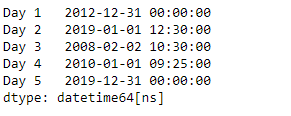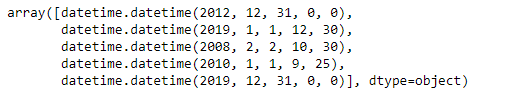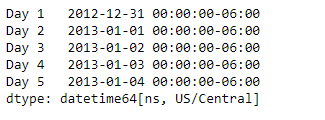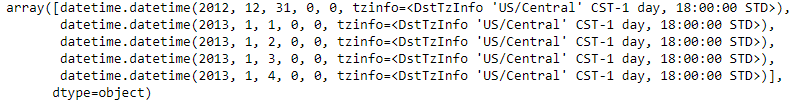# Python | Pandas Series.dt.to_pydatetime

`Series.dt` can be used to access the values of the series as datetimelike and return several properties. Pandas` Series.dt.to_pydatetime()` function return the data as an array of native Python datetime objects. Timezone information is retained if present.

Syntax: Series.dt.to_pydatetime()

Parameter : None

Returns : numpy.ndarray

Example #1: Use `Series.dt.to_pydatetime()` function to return the given series object as an array of native python datetime object.

 `# importing pandas as pd ` `import` `pandas as pd ` ` `  `# Creating the Series ` `sr ``=` `pd.Series([``'2012-12-31'``, ``'2019-1-1 12:30'``, ``'2008-02-2 10:30'``, ` `               ``'2010-1-1 09:25'``, ``'2019-12-31 00:00'``]) ` ` `  `# Creating the index ` `idx ``=` `[``'Day 1'``, ``'Day 2'``, ``'Day 3'``, ``'Day 4'``, ``'Day 5'``] ` ` `  `# set the index ` `sr.index ``=` `idx ` ` `  `# Convert the underlying data to datetime  ` `sr ``=` `pd.to_datetime(sr) ` ` `  `# Print the series ` `print``(sr) `

Output :Now we will use `Series.dt.to_pydatetime()` function to return the data as an array of native Python datetime objects.

 `# return the series data as a  ` `# native python datetime data ` `result ``=` `sr.dt.to_pydatetime()  ` ` `  `# print the result ` `print``(result) `

Output :As we can see in the output, the `Series.dt.to_pydatetime()` function has successfully returned the underlying data of the given series object as an array of native python datetime data.

Example #2 : Use `Series.dt.to_pydatetime()` function to return the given series object as an array of native python datetime object.

 `# importing pandas as pd ` `import` `pandas as pd ` ` `  `# Creating the Series ` `sr ``=` `pd.Series(pd.date_range(``'2012-12-31 00:00'``, periods ``=` `5``, freq ``=` `'D'``, ` `                            ``tz ``=` `'US / Central'``)) ` ` `  `# Creating the index ` `idx ``=` `[``'Day 1'``, ``'Day 2'``, ``'Day 3'``, ``'Day 4'``, ``'Day 5'``] ` ` `  `# set the index ` `sr.index ``=` `idx ` ` `  `# Print the series ` `print``(sr) `

Output :Now we will use `Series.dt.to_pydatetime()` function to return the data as an array of native Python datetime objects.

 `# return the series data as a  ` `# native python datetime data ` `result ``=` `sr.dt.to_pydatetime()  ` ` `  `# print the result ` `print``(result) `

Output :As we can see in the output, the `Series.dt.to_pydatetime()` function has successfully returned the underlying data of the given series object as an array of native python datetime data.

My Personal Notes arrow_drop_upCheck out this Author's contributed articles.

If you like GeeksforGeeks and would like to contribute, you can also write an article using contribute.geeksforgeeks.org or mail your article to contribute@geeksforgeeks.org. See your article appearing on the GeeksforGeeks main page and help other Geeks.

Please Improve this article if you find anything incorrect by clicking on the "Improve Article" button below.

Article Tags :

Be the First to upvote.

Please write to us at contribute@geeksforgeeks.org to report any issue with the above content.